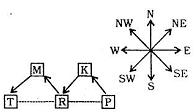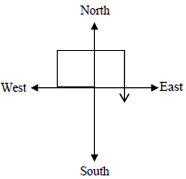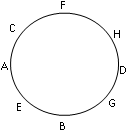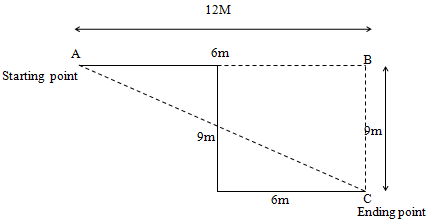# Maruti Reasoning Question

1. K is a place which is located 2 km away in the north-west direction from the capital P. R is another place that is located 2 km away in the south-west direction from K. M is another place and that is located 2 km away in the north-west direction from R. T is yet another place that is located 2 km away in the south-west direction from M. In which direction is T located in relation to P?
1. South-west
2. North-west
3. West
4. NorthIt is clear that T is located to the West of P.
2. One evening, Raja started to walk toward the Sun. After walking a while, he turned to his right and again to his right. After walking a while, he again turned right. In which direction is he facing?
1. South
2. East
3. West
4. NorthHence he is facing south.
DIRECTIONS for questions 3 to 5 : In these questions, relationships between different elements are shown in the statements. These statements are followed by two conclusions.
Give answer (A): if only conclusion I follows
Give answer (B): if only conclusion II follows
Give answer (C): if either I or II follows
Give answer (D): if neither I nor II follows
Give answer (E): if both I and II follow
1. Statements: N ≥ O ≥ P = Q >R
Conclusions: I. N>R II. R = N
1. 1
2. 2
3. 3
4. 4
5. 5
As N ≥ O ≥ P = Q > R
⇒ N > R , So 1st Conclusion is correct.
⇒ As R is less than Q then clearly R is not equal to N.
Therefore, Only conclusion I follows.
2. Statements: W ≤ X < Y = Z > A; W< B Conclusions: I. B > Z II. W < A
1. 1
2. 2
3. 3
4. 4
5. 5
I. We have B > W and Z > W
On this basis, we cannot conclude that B > Z
II. Here, X > W and X < Z > A
On this basis, we cannot conclude that W < A
Therefore, none of the conclusions follows.
3. Statements: H > I > J >K; L < M< K Conclusions: I. I > M II. L< H
1. 1
2. 2
3. 3
4. 4
5. 5
I. Here, Z > X > W
⇒Z > W and also, B > W
On this basis, we cannot clearly determine the relation between B and Z.
II. Z > X > W
⇒Z > W and also, Z > A
Again, we cannot conclude that W < A
Therefore, none of the conclusion follows.
DIRECTIONS for questions 6 to 8 :Study the given information carefully and answer the given questions:
Eight people -A, B, C, D, E, F, G and H are sitting around a circular table facing the centre, not necessarily in the same order. Three people are sitting between A and D. B is sitting second to the right of A. C is to the immediate right of F. D is not an immediate neighbour of either F or E. H is not an immediate neighbour of B.
4. What is E's position with respect to G?
1. Third to the left
2. Second to the right
3. Third to the right
4. Second to the left
5. Fifth to the rightFrom above figure it is clear that E is sitting 2nd to the left of G.
So, the correct answer is option D.
5. Four of the following five are alike in a certain way, based on their seating positions in the above arrangement and so form a group. Which one does not belong to the group?
1. GE
2. DC
3. AF
4. AB
5. CEFrom above figure it is clear that the answer is 2nd option, As the gap between the persons in all other pairs is of 1 person.
Therefore, the correct answer is option B.
6. Who is sitting third to the right of the one who is sitting to the immediate right of H?
1. A
2. B
3. E
4. C
5. GFrom above figure it is clear that answer is 3rd option.
7. How many such digits are there in the number 3246759, each of which will occupy the same position when rearranged in ascending order as in the number?
1. None
2. One
3. Two
4. Three
5. More than three